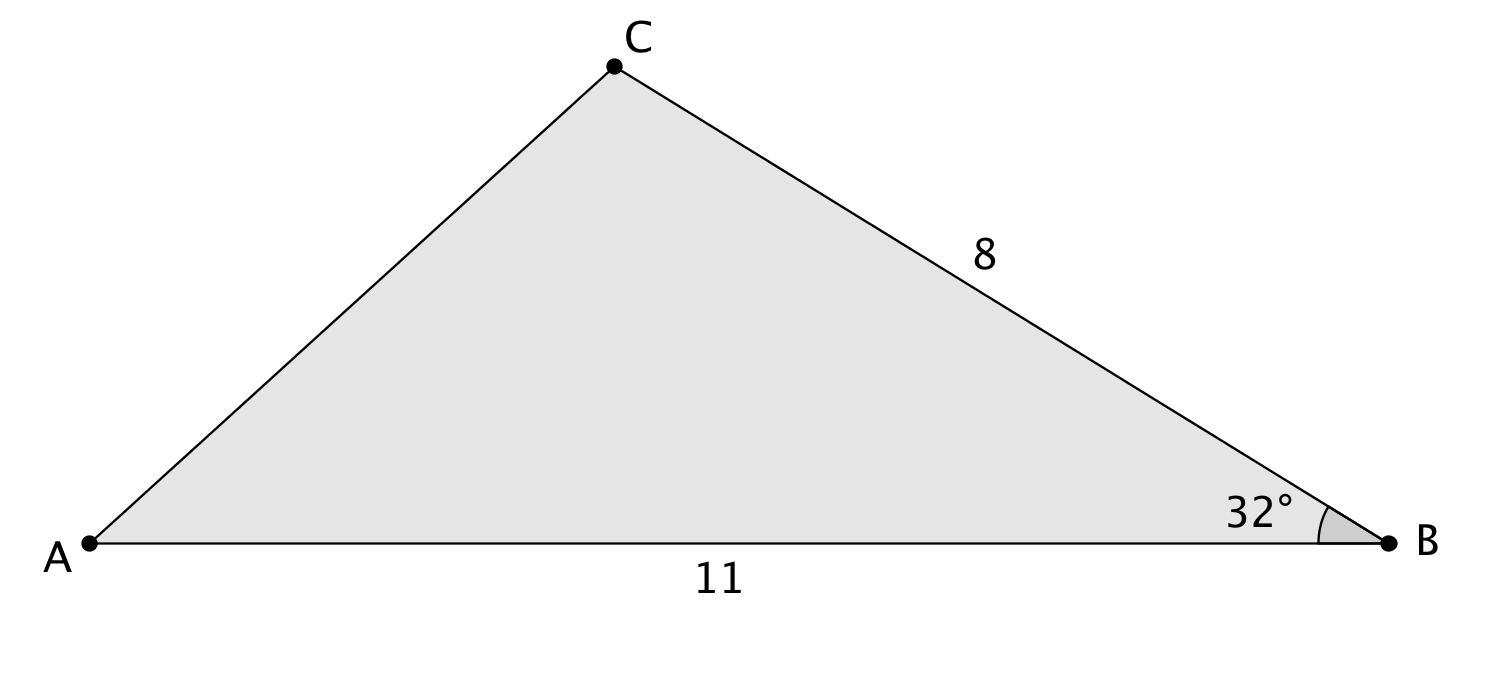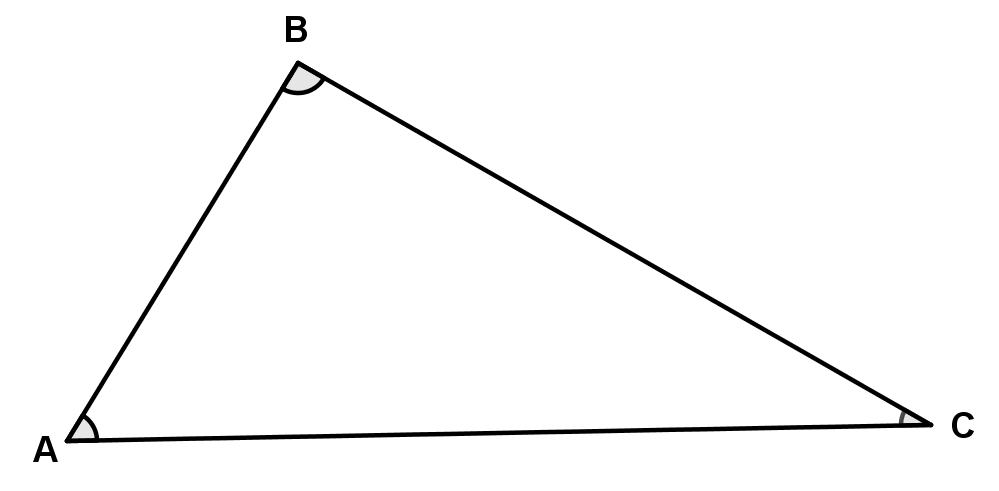# Right Triangles and Trigonometry

## Objective

Derive the area formula for any triangle in terms of sine.

## Common Core Standards

### Core Standards

?

• G.SRT.D.9 — Derive the formula A = 1/2 ab sin(C) for the area of a triangle by drawing an auxiliary line from a vertex perpendicular to the opposite side.

?

• 6.G.A.1

## Criteria for Success

?

1. Use the area formula for triangles to determine missing information in non-right triangles.
2. Identify that an auxiliary altitude is necessary in non-right triangles to represent the height.
3. Write the measurement of the height of a non-right triangle in terms of sine of the angle opposite to the height.
4. Assign the variables $A$, $B$, and $C$ to each of the vertices of a non-right triangle.
5. Assign the variables $a$$b$, and $c$ to each side opposite vertices $A$, $B$, and $C$ respectively in non-right triangles.
6. Generalize the area formula for non-right triangles as $\mathrm{Area}=\frac{1}{2}ab\mathrm{sin}(C)$.
7. Verify that the formula works for any non-right triangle once $a$$b$, and $c$  sides and $A$, $B$, and $C$ angles are defined.

## Tips for Teachers

?

This post on Math Central is helpful in developing knowledge on the area formula in terms of sine for triangles.

## Anchor Problems

?

### Problem 1

Find the area of the following triangle.### Problem 2

Write a formula for the area of this non-right triangle.## Problem Set

?

The following resources include problems and activities aligned to the objective of the lesson that can be used to create your own problem set.

• Include problems where the students are given the area and need to find missing information about the non-right triangle.

Explain why ${\frac{1}{2}ab\mathrm{sin}(C)}$ gives the area of a triangle with sides $a$ and $b$ and included angle $C$.